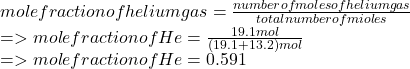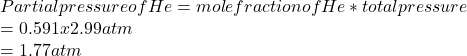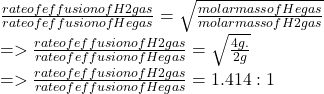## A mixture of gases at 2.99 atm can You have two gases, and , at the same temperature. Determine the ratio of effusion rates of and .ists of

Question

A mixture of gases at 2.99 atm can You have two gases, and , at the same temperature. Determine the ratio of effusion rates of and .ists of 13.2 moles of hydrogen gas and 19.1 moles of helium gas. Determine the partial pressure of the helium gas.

in progress 0
6 months 2021-07-17T06:55:41+00:00 1 Answers 14 views 0

Given total pressure of the gas mixture(hydrogen and helium) is 2.99 atm

Number of moles of hydrogen is —- 13.2 mol

Number of moles of helium is —- 19.1 mol

Determine the partial pressure of the helium gas.

Ratio of effusion rates of the two gases.

Explanation:

According to Dalton’s law of partial pressures,

partial pressure of a component gas in a mixture is:

partial pressure of a gas = total pressure x mole fraction

mole fraction of helium gas is:Partial pressure of He gas is:Effusion rate of a gas is inversely proportional to its square root of its molecular mass.Hence, rates of effusion of H2:He is 1.414:1.